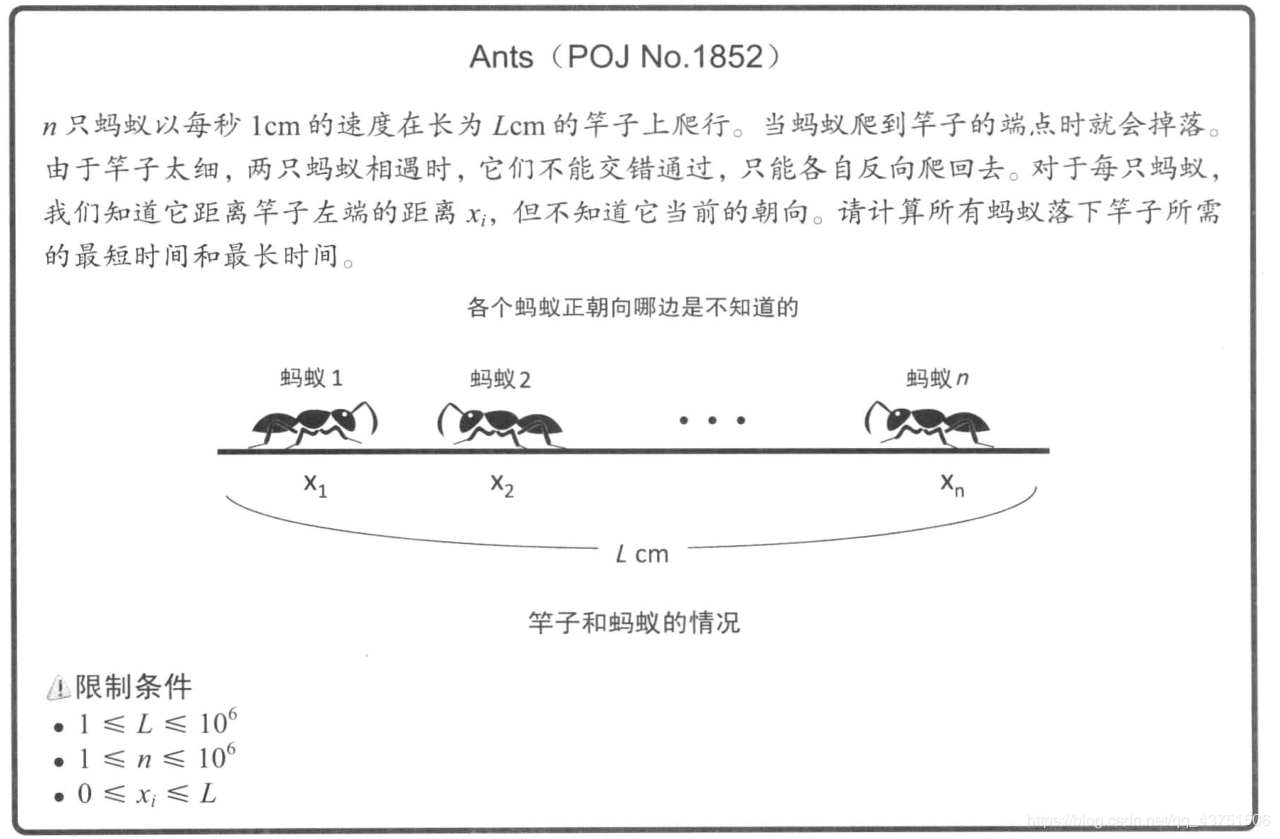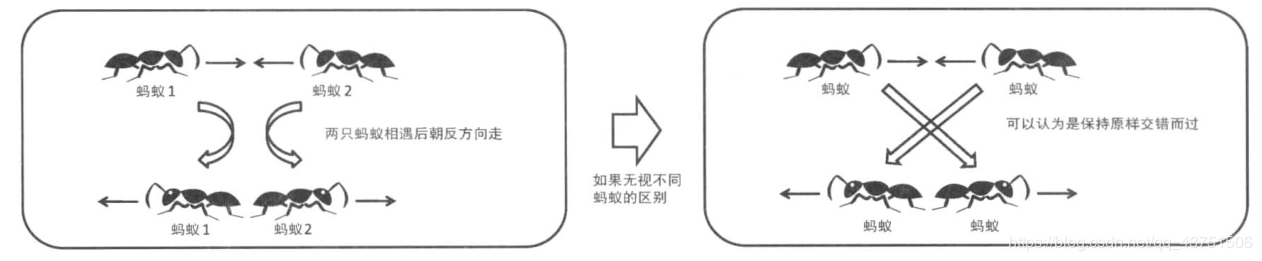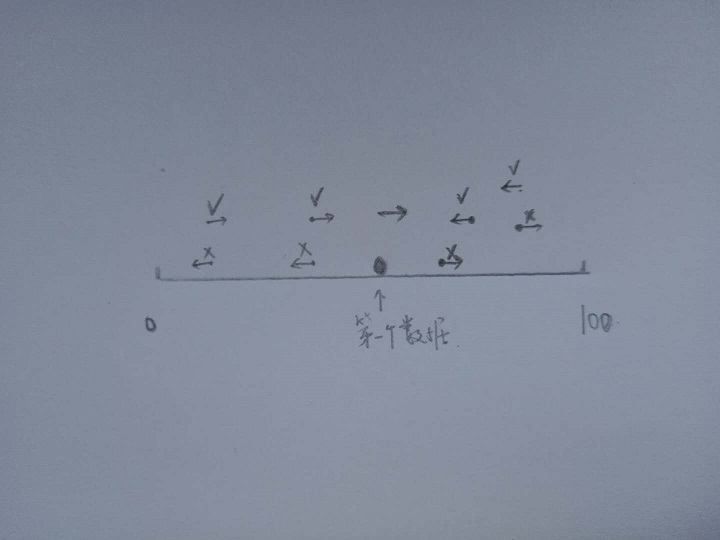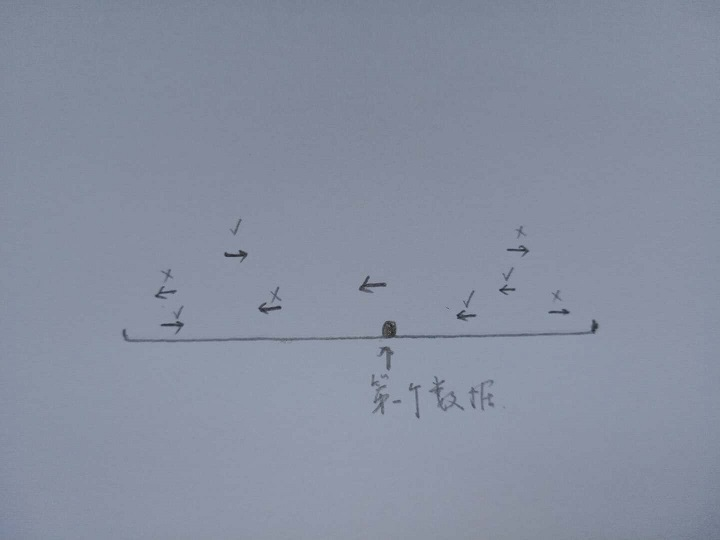# 蓝桥杯 试题 历届试题 蚂蚁感冒

长100厘米的细长直杆子上有n只蚂蚁。它们的头有的朝左，有的朝右。

每只蚂蚁都只能沿着杆子向前爬，速度是1厘米/秒。

当两只蚂蚁碰面时，它们会同时掉头往相反的方向爬行。

这些蚂蚁中，有1只蚂蚁感冒了。并且在和其它蚂蚁碰面时，会把感冒传染给碰到的蚂蚁。

请你计算，当所有蚂蚁都爬离杆子时，有多少只蚂蚁患上了感冒。

第一行输入一个整数n (1 < n < 50), 表示蚂蚁的总数。

接着的一行是n个用空格分开的整数 Xi (-100 < Xi < 100), Xi的绝对值，表示蚂蚁离开杆子左边端点的距离。正值表示头朝右，负值表示头朝左，数据中不会出现0值，也不会出现两只蚂蚁占用同一位置。其中，第一个数据代表的蚂蚁感冒了。

要求输出1个整数，表示最后感冒蚂蚁的数目。

3
5 -2 8

1

5
-10 8 -20 12 25

3```//输入
int n,L;
int x[Max_N];//保存蚂蚁的位置

void solve()
{
int Min_T = 0, Max_T = 0;

for(int i=0; i<n; i++)
{
Min_T = max( Min_T, min(x[i],L-x[i])); //计算最短时间
Max_T = max( Max_T, max(x[i],L-x[i]));//计算最长时间
}
printf("%d %d\n",Min_T,Max_T);
}```

• 第一个数据为正值( 第一只感冒的蚂蚁头朝右 )• 再看第一个数据为负值（即第一只感冒的蚂蚁头朝左）```#include<cstdio>

//其实只要求在感冒位置左边方向向右;右边方向向左的个数就可以了

const int Max_N = 50;

int n;
int x[Max_N+1];// 0:没有蚂蚁 -1:向左  1:向右

int ant; //感冒蚂蚁的位置

void solve()
{
int res = 0;
for(int i=1; i<ant; i++){//左边 向右
if( x[i]==1 )    res++;
}
for(int i=ant+1; i<=100; i++){//右边 向左
if( x[i]==-1 )    res++;
}
printf("%d\n",res+1);//加上原来的第一只感冒的蚂蚁
}

int main()
{
scanf("%d",&n);
int a;
scanf("%d",&a);
ant = a>0 ? a : -a;//第一个位置是感冒的位置
while( --n )
{//剩下的n-1个
scanf("%d",&a);
if( a>0 )    x[a] = 1;//向右
else    x[-a] = -1; //向左
}

solve();

return 0;
}```